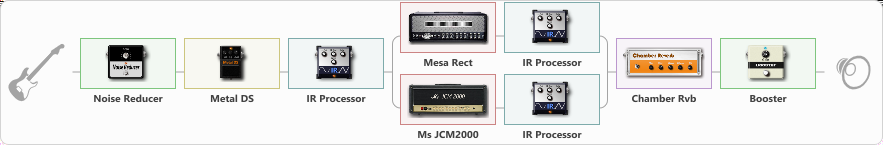# August Burns Red (Messenger)

Discussion in 'ToneLib-GFX presets' started by Kcote204, Feb 6, 2022.

1. August Burns Red (Messenger)

Preset name: August Burns Red

Effects chain:Effect: "Noise Reducer" (Dynamique / Filtre), active - "yes"
{
"Sens" = 93
"Mode" = Soft
}

Effect: "Metal DS" (Overdrive / Distortion), active - "yes"
{
"Dist" = 49
"Bass" = 35
"Middle" = 55
"Treble" = 51
"Level" = 51
}

Effect: "IR Processor" (Cabinets), active - "yes"
{
"IR" = pre-tonal_dc
"Low Cut (Hz)" = 204
"Hi Cut (kHz)" = 17.0
"Mix" = 50
"Level (dB)" = 2
}

Effect: "Splitter" (Dynamique / Filtre)
{
"A-Bypass" = Off
"A-Pan" = 100
"A-Level" = 55
"B-Bypass" = Off
"B-Pan" = -100
"B-Level" = 55

'A' branch:
{

Effect: "Mesa Rect" (Simulateurs d'amplis), active - "yes"
{
"Gain" = 75
"Bass" = 39
"Middle" = 49
"Treble" = 57
"Presence" = 84
"Master" = 74
"Level (dB)" = 0
}

Effect: "IR Processor" (Cabinets), active - "yes"
{
"IR" = charv.1_dc
"Low Cut (Hz)" = 0
"Hi Cut (kHz)" = 20.0
"Mix" = 100
"Level (dB)" = 0
}
}
'B' branch:
{

Effect: "Ms JCM2000" (Simulateurs d'amplis), active - "yes"
{
"Gain" = 75
"Bass" = 30
"Middle" = 50
"Treble" = 51
"Presence" = 82
"Master" = 68
"Level (dB)" = 0
}

Effect: "IR Processor" (Cabinets), active - "yes"
{
"IR" = ibanez_dc
"Low Cut (Hz)" = 0
"Hi Cut (kHz)" = 17.0
"Mix" = 100
"Level (dB)" = -1
}
}
}

Effect: "Chamber Rvb" (Réverbération), active - "yes"
{
"Time" = 1.5
"PreDelay" = 10
"LoDamp" = 20
"HiDamp" = 25
"Mix" = 15
}

Effect: "Booster" (Dynamique / Filtre), active - "yes"
{
"Gain" = 60
}

Note: You will need to download and install the ToneLib-GFX software to use the preset.

File size:
67.6 KB
Views:
1,971